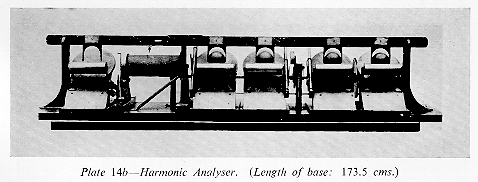## Fourier analysis of the tidal recordThe Tidal Analyzer, (Kelvin, opposite p. 304).

Once the working hypothesis is established, that the astronomical tidal function for any given port is a sum of a certain number of constituents whose frequencies are known a priori then the amplitudes and phases of the constituents may be determined by Fourier analysis.

To put the sum in more standard form, a constituent Hcos(vt + p) will be rewritten using a standard trigonometric identity as Acosvt + Bsinvt (with A = Hcosp and B = -Hsinp).

There are three facts about sine and cosine finctions that make Fourier analysis work.

First fact. In the long run, the average value of any function of the form sin(vt) or cos(vt) must be zero. This is clear from looking at the graphs of these functions: each positive contribution to the average is exactly cancelled by a negative one.

Second fact. For different speeds v and w the average value of the product

cos(vt) cos(wt)
goes to zero as the average is taken over longer and longer time intervals. The reason is that in the long run the times when the two functions are out of phase (so the product is negative) will cancel the contributions from the times they are in phase. Similarly for the products
cos(vt) sin(wt),
cos(vt) sin(vt),
sin(vt) sin(wt).

Third fact. The average value of the products

cos(vt) cos(vt),
sin(vt) sin(vt)

goes to exactly 1/2 if the averages are taken over longer and longer time intervals. First of all, in each case the two factors are always in phase, in fact equal, so their product is always either the square of a positive number or the square of a negative number, or zero, but in any case never negative, so there can be no cancellation. Why is the average exactly 1/2? Since the graphs of the sine function and the cosine function are so similar, we can expect that in the long run sine-squared and cosine-squared would have the same average. On the other hand the basic trigonometric identity

sine-squared + cosine-squared = 1

which holds everywhere must hold for the averages as well. Since the two averages are equal, and add up to one, they must each equal 1/2. Click here for a more standard formulation, where the averages are defined as integrals.

Suppose, to take a simple example, that the tidal curve from an ideal port was exactly

A(t) = A0 + A1 cos(vt) + B1 sin(vt) + A2 cos(wt) + B2 sin(wt),

where we know the ``speeds'' v and w, and we want to calculate the coefficients A0, A1, A2, B0, B1, B2.

A0. Since by ``Fact one'' each of the sine and cosine terms averages out to zero in the long run, the long-term average of the tidal height function must be exactly A0.

A1. We multiply A(t) by cos(vt) and measure the average value of the product over a long time interval. There are five terms:
A0cos(vt) has average zero by ``Fact one.''
A1cos(vt)cos(vt) has average (1/2) A1 by ``Fact three.''
B1sin(vt)cos(vt)
A2cos(wt)cos(vt)
B2sin(wt)cos(vt) all have average 0 by ``Fact two.''

So multiplying A(t) by cos(vt) and averaging over a long time period produces 1/2 the coefficient A1.

B1. The same phenomenon happens when we multiply A(t) by sin(vt) and take a long-term average. This time:
A0sin(vt) has average zero by ``Fact one.''
A1cos(vt)sin(vt) has average 0 by ``Fact two.''
B1sin(vt)sin(vt) has average (1/2) B1 by ``Fact three''
A2cos(wt)sin(vt)
B2sin(wt)sin(vt) both have average 0 by ``Fact two.''

A2, B2 are obtained in the same way: multiply by cos(wt) or sin(wt), take a long-term average, and multiply by 2 (to get rid of the 1/2's).

What we just did for five terms works just as well for fifty: if R(t) is the record from a tide gauge at the port in question, the cosine and sine amplitudes A and B for a particular speed v may be determined by

A = twice the long-term average of R(t)cos(vt)
B = twice the long-term average of R(t)sin(vt).

Kelvin devised machines to compute these averages for some values of v. The Tidal Analyzer (see above) was 20 feet long and used 11 coupled sphere-cylinder-disk integrators to mechanically compute the average height of the tide and the sine and cosine coefficients for the first 5 constituents. I believe this machine no longer exists, but a working model (``The Harmonic Analyzer'') designed to calculate the coefficients in the expression

A + Bsint+ Ccost+ Dsin2t+ Ecos2tThe Harmonic Analyzer (Green & Lloyd, p. 46).

is in the Kelvin Museum in Glascow.

Back to Main Tide page.